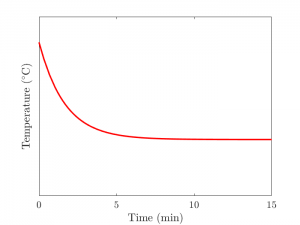You might wonder why your coffee cools down more in the first 5 minutes after you’ve made it than it does in the next 5 minutes. When losing heat, the coffee undergoes a process called diffusion that makes it try to get closer to the surrounding temperature. But how long does it take the coffee to get from piping hot, fresh-off-the-press to lukewarm, room temperature?Figure 1: Example of the temperature of a cup of coffee approaching room temperature

Mathematically, this process takes an infinite amount of time for the system to become exactly equal to its equilibrium. However, this is not a very useful answer for scientists, engineers, or even baristas to try and consider. So, how can we discuss the transition time—that is; how do we know how long it takes to get to its final temperature?

This research project aimed to extend an approach for diffusion processes (where something like heat is simply transmitted around to nearby particles) to diffusion-decay processes, where a proportion of the quantity in question present is lost over time as well. Using a formula for a quantity called ‘moments’, the research managed to successfully determine a formula for the time to equilibrium that relied only on solving a simpler, iterated differential equation.

The advantage of this research is that it removes one of the biggest roadblocks to finding this transition time: calculating solutions to partial differential equations can be an arduous process—even when using computers. However, if the approach with the moments is taken, this roadblock is bypassed, and a much simpler problem can be solved instead to find the transition time.

There is an extra benefit to extending the method to a diffusion-decay problem: solutions to equilibrium problems governed by coupled diffusion, where there are two different equations whose behaviours rely on each other. These problems can be broken down into two separated diffusion-decay problems. It may be possible in future developments of this procedure to find the overall transition time for such coupled diffusion problems.

Jonah Klowss
Queensland University of TechnologyView Jonah Klowss
Profile